# Secret Investigations: Revelations WalkthroughSecret Investigations: Revelations Walkthrough
* OS: Windows 7 / Vista / XP
* CPU: Pentium 3 1.0 GHz or HigherSecret Investigations: Revelations Walkthrough
* OS: Windows 7 / Vista / XP
* CPU: Pentium 3 1.0 GHz or Higher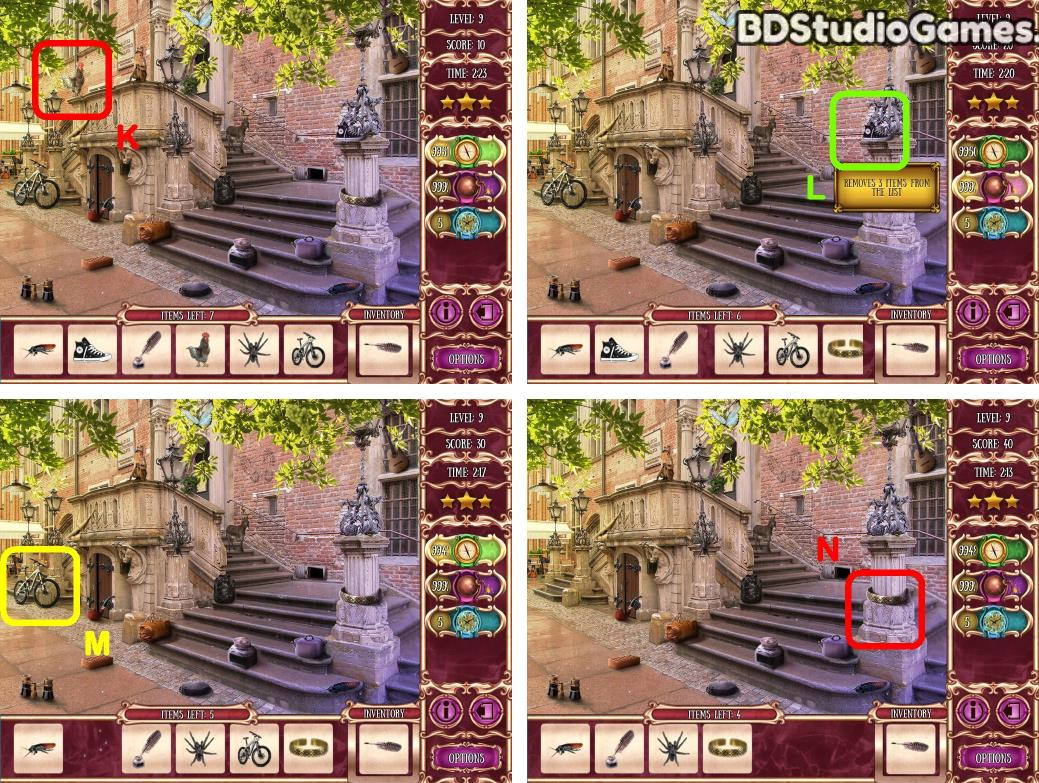Take it. (K)
Take it. (L)
Take it. (M)
Take it. (N)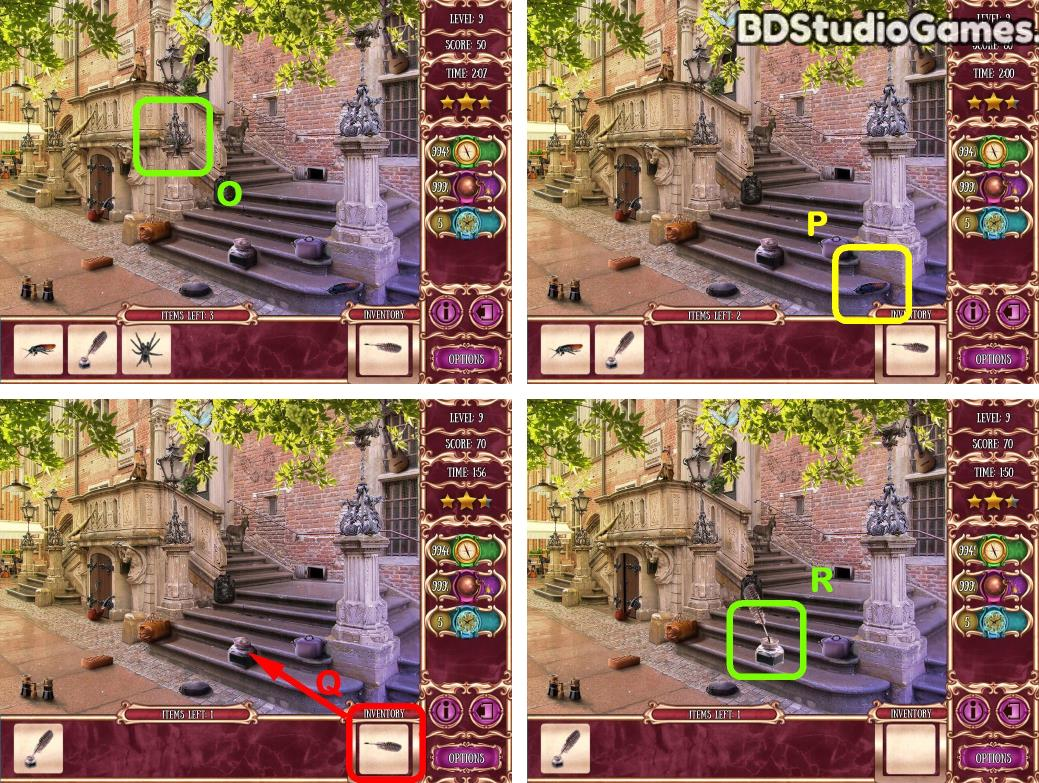Take it. (O)
Take it. (P)
Use object on here. (Q)
Take it. (R)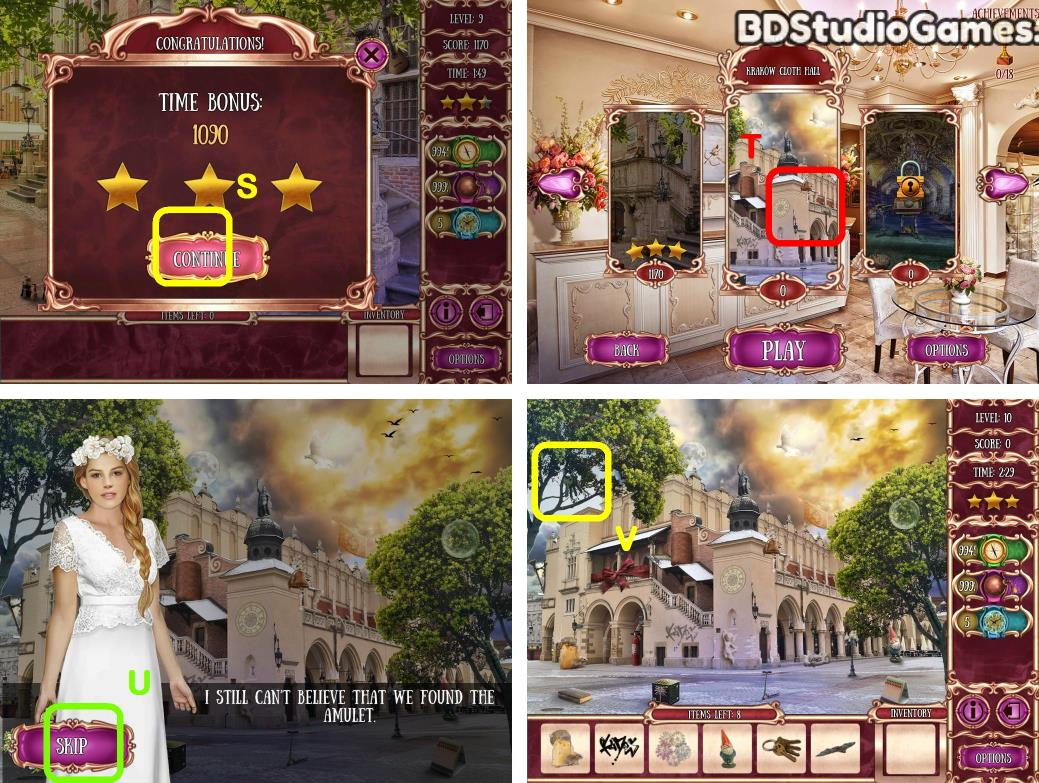Take it. (V)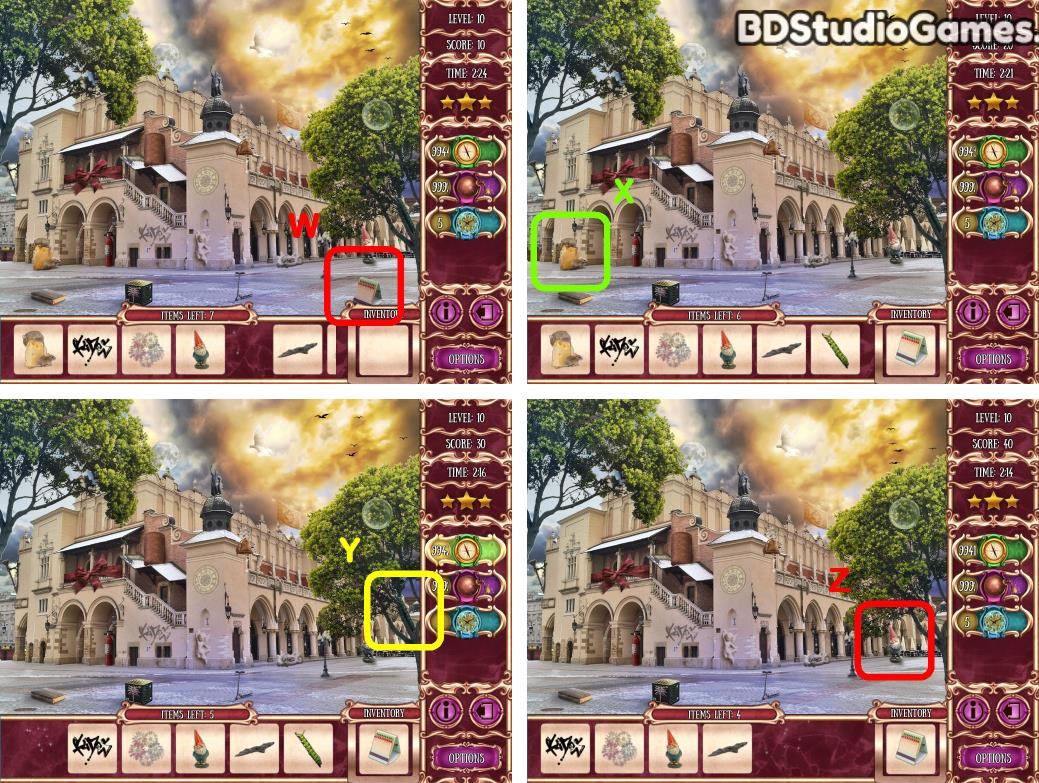Take it. (W)
Take it. (X)
Take it. (Y)
Take it. (Z)Take it. (A)
Take it. (B)
Use object on here. (C)
Take it. (D)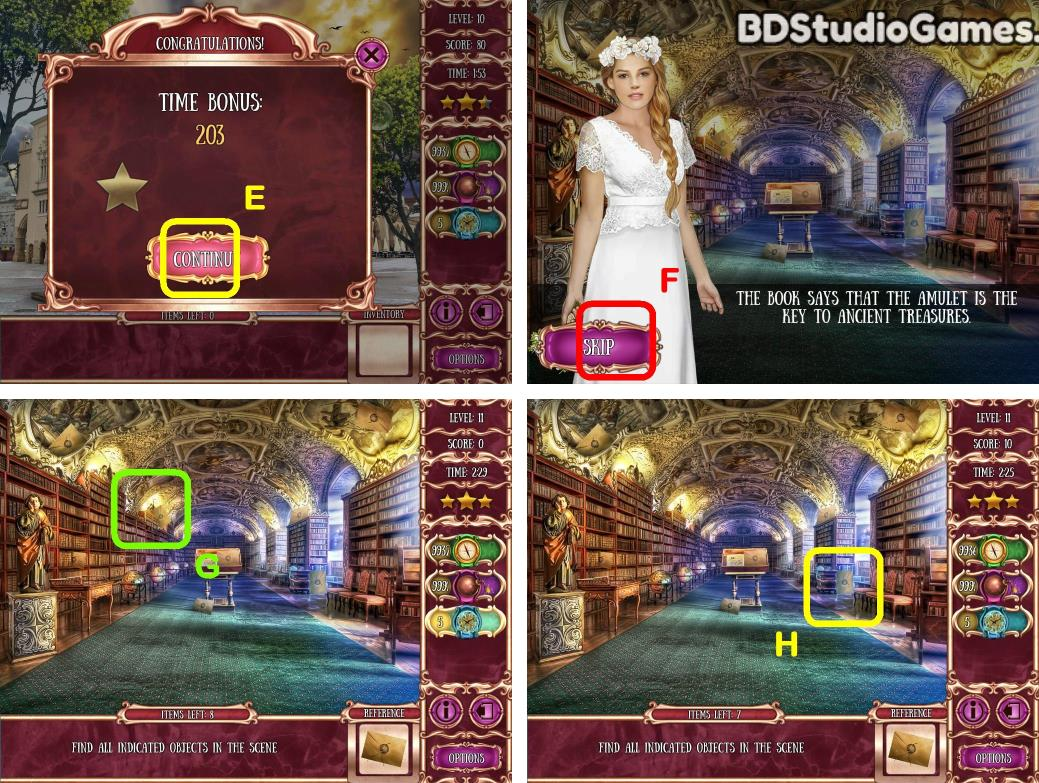Take it. (G)
Take it. (H)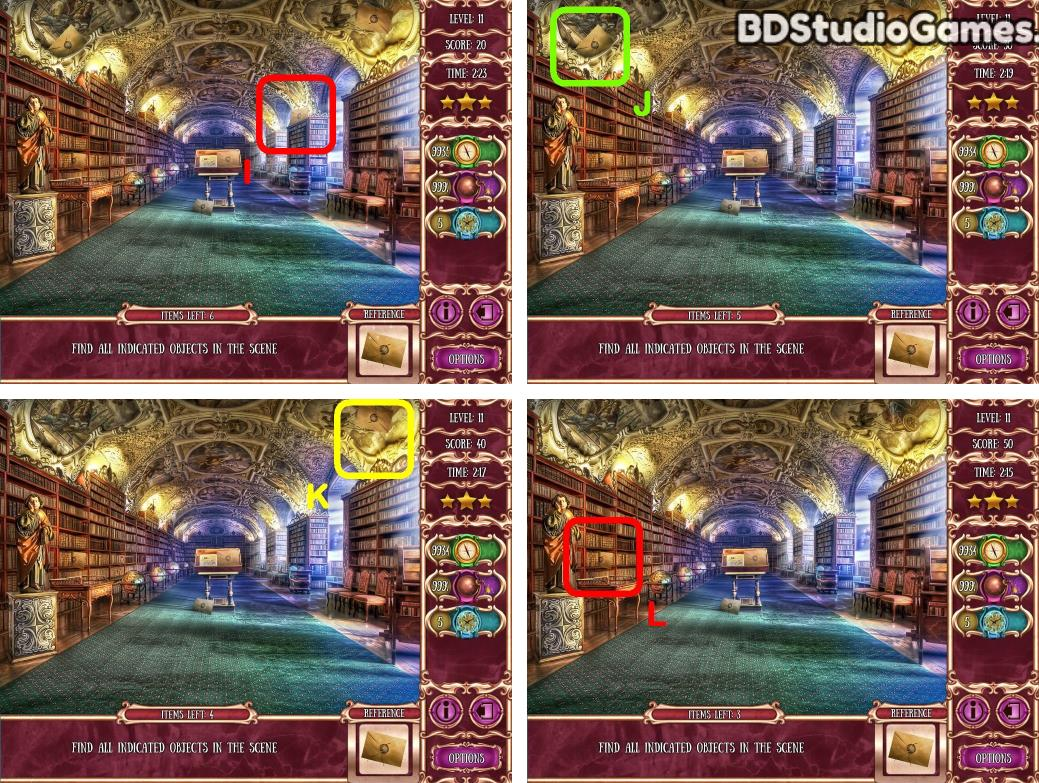Take it. (I)
Take it. (J)
Take it. (K)
Take it. (L)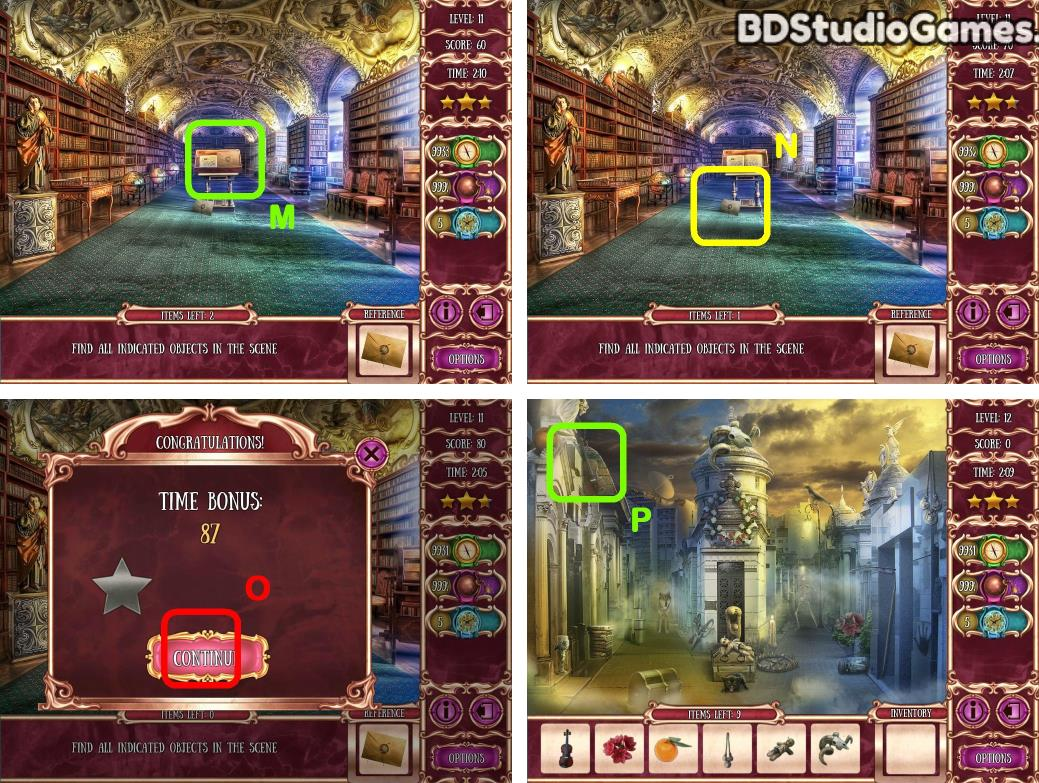Take it. (M)
Take it. (N)
Take it. (P)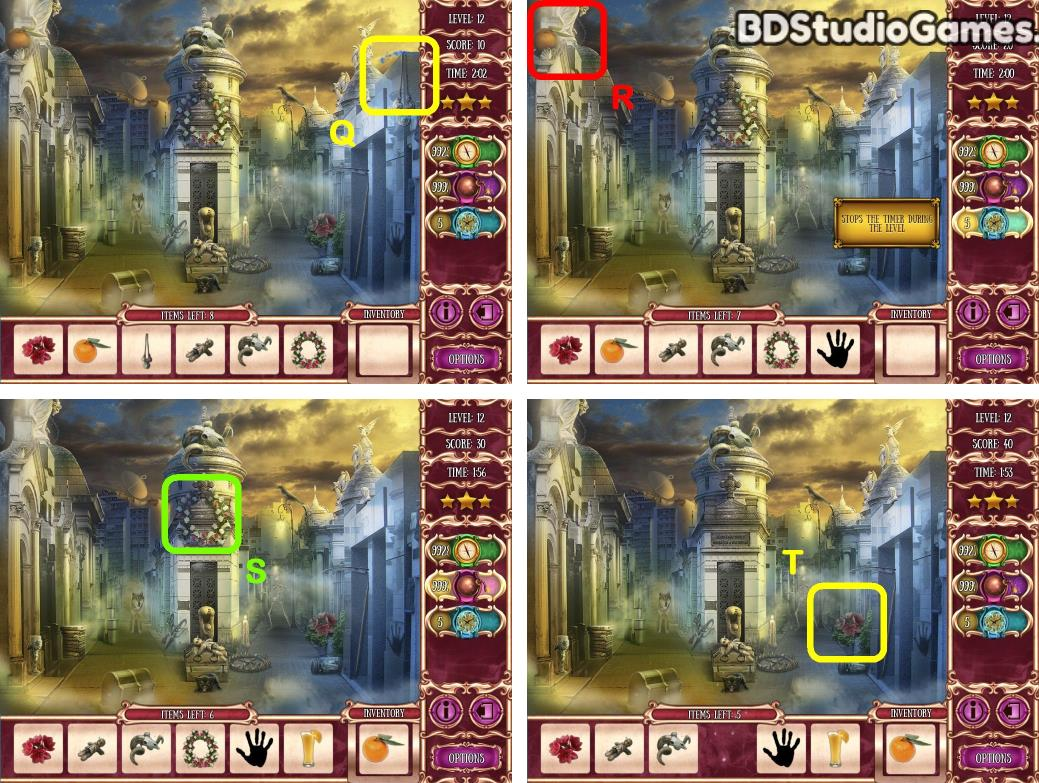Take it. (Q)
Take it. (R)
Take it. (S)
Take it. (T)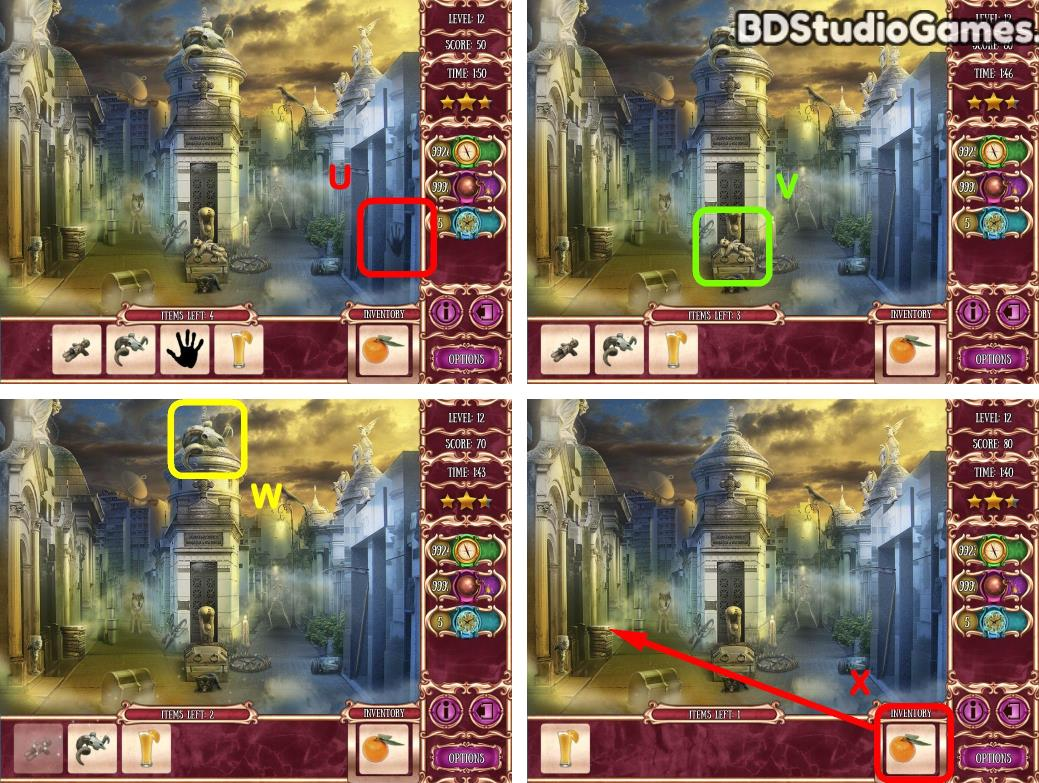Take it. (U)
Take it. (V)
Take it. (W)
Use object on here. (X)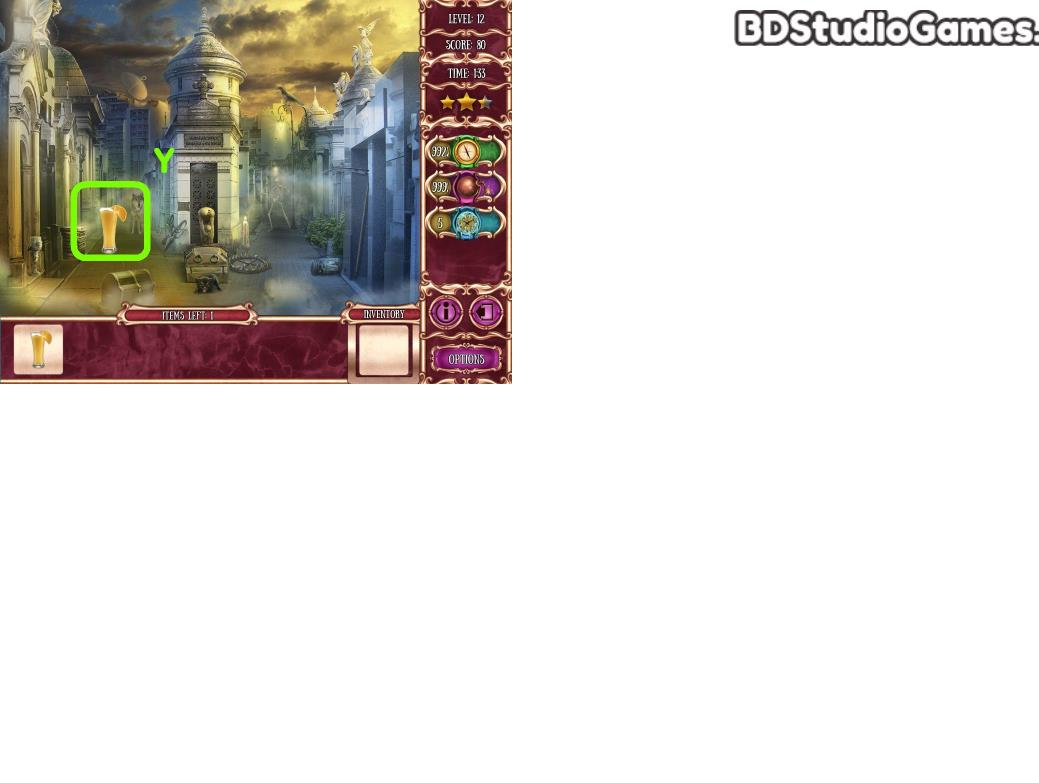Take it. (Y)